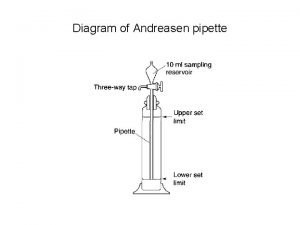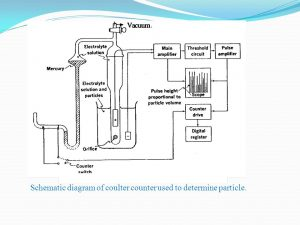# Micromeretics and powder rheology: Methods of determining particle size and volume – Sedimentation and Coulter Counter method and MCQs for GPAT, NIPER, Pharmacist and Drug Inspector exam

## Micromeretics and powder rheology: Methods of determining particle size and volume – Sedimentation and Coulter Counter method and MCQs for GPAT, NIPER, Pharmacist and Drug Inspector exam

### Sedimentation Technique:

Equivalent diameter: Stokes’ diameter; frictional drag diameter—a sphere having an equivalent drag force to a particle of the same diameter in the same fluid at the same velocity.

Range of analysis: 1 μm to about 200 μm.

It follows Stoke’s Law.

Stoke’s Law:

V = d2g(Ƿps) / 18ƞ

Methodology:

1.      Allow a homogeneous suspension to settle in a cylinder, take samples from the settling suspension at a fixed horizontal level at intervals of time.

2.       Each sample will contain a representative sample of the suspension, with the exception of particles greater than a critical size, all of which will have settled below the level of the sampling point.

3.      The concentration of solid in a sample taken at time t is determined.

4.       This concentration expressed as a percentage of the initial concentration gives the percentage (w/w) of particles whose falling velocities are equal to or less than x/t. Substitution in the equation above gives the corresponding Stokes’ diameter.Fig 1 – Andreasen pipette apparatus for size determination by sedimentation (taken from micromeretics slideshare)

Table 1 – Representation of weight distribution values obtained by the sedimentation method

 Time (s) Height (cm) Particle diameter calculated by Stokes’ equation (µm) Weight of residue (g) Weight of residue (%) Cumulative weight of residue (%) Log particle size 120 20.0 44.5 As weighed – – 1.65 240 19.6 30.5 As weighed – – 1.5

1. The apparatus is inexpensive and the technique is simple.
2. The results obtained are precise, provided the technique is adequately standardized.

1. The method is laborious as separate analyses are required for each experimental point on the distribution curve.
2. Very small particles subjected to Brownian motion cannot be determined accurately due to prolonged settling rates.

Coulter Counter Method (Particle Volume Measurement):

Equivalent diameter: Volume diameter.

Range of analysis: 0.1 μm to about 1000 μm.

Methodology:

Coulter counter is a popular instrument to determine particle volume and particle size based on the conductivity measurement. It operates on the principle that when a particle suspended in a conducting liquid passes through a small orifice (opening), on either side of which are electrodes, a change in electric resistance occurs. The change in electric resistance is proportional to the volume of the particle.

Figure 2 shows the mechanical parts of the instrument. It consists of two electrodes: one is dipped into a beaker containing the particle suspension in an electrolyte (such as 0.9% NaCl) and the other is dipped into the electrolyte solution contained in a glass tube, which in turn is immersed into the beaker containing the particle suspension in the electrolyte. The glass tube has a very small aperture at its lower end through which the particles are sucked into the inner glass tube.Fig  2 – Representation of Coulter counter apparatus (taken from Industrial Pharmacy slide player)

For the experiment, powder samples are suspended in the electrolyte to form a very dilute suspension. The particle concentration is arranged so that only one particle travels through the aperture. A constant voltage is applied across the electrodes so as to produce a current. As the particle passes through the aperture, it displaces its own volume of electrolyte and changes the resistance between the electrodes, which causes a pulse in the voltage. The magnitude of pulse will be proportional to the volume of the particle. The changes in voltage are amplified and impulses above a predetermined threshold value are counted, so that the recorder provides a count of the number of particles over a certain size.

1. Results are expressed as particle volume, and hence the diameter of the sphere of equivalent volume can be easily calculated.
2. Process is rapid with a single count taking less than 30 s.
3. Results are reliable since large numbers of particles are counted.
4. Operational simplicity reduces operator variables, enabling reproducible results.

1. The material has to be suspended in an electrolyte liquid before measurement.
2. Aggregation of particles can give false results.

Multiple choice questions (MCQs)

1.Which of the following diameters is obtained by sedimentation technique?

a)stokes diameter

b)martins diameter

c)ferrets diameter

d)projected diameter

2.Range of analysis by sedimentation technique is

a)1 μm to 200 μm

b)1 nm to 200 nm

c)10 nm to 100 nm

d)1 nm to 10 nm

3.Sedimentation Technique follows which of the following law?

b)stokes law

c)daltons law

d)rittinger law

4.Stoke’s Law is given by which of the following equation?

a)V = d2g(Ƿps) / 18

b)V = d2g(Ƿps) / ƞ

c)V = dg(Ƿps) / 18ƞ

d)V = d2g(Ƿps) / 18ƞ

5.Which of the following apparatus is used in sedimentation technique?

a)stalagmometer

b)pycnometer

c)andresen pipette

d)viscometer

6.Andreasen pipette consist of

a)sampling reservoir

b)pipette

c)three way tap

d)all of the above

7.Which of the following are advantages of sedimentation technique?

a)The apparatus is inexpensive and the technique is simple

b)The results obtained are precise, provided the technique is adequately standardized

c)The method is laborious as separate analyses are required for each experimental point on the distribution curve

d)a) and b)

8.Which of the following are disadvantages of sedimentation method?

a)The method is laborious as separate analyses are required for each experimental point on the distribution curve

b)Very small particles subjected to Brownian motion cannot be determined accurately due to prolonged settling rates

c)both of these

d)none of these

a)Particle size(aerosols)

b)Particle volume

c)Particle size

d)All of the above

10.Laser diffraction is used for

a)Particle volume

b)Particle size

c)Both

d)None of these

11.Coulter Counter Method is used for the determination of

a)particle shape

b)particle size

c)particle volume

d)surface area

12.Which of the following equipment is used for determining the particle size distribution of pharmaceutical dispersions?

a)Differential scanning calorimeter

b)Sorptometer

c)Andreasen pipette

d)Shear box

13.The rate of sedimentation of particles is directly proportional to

a)Particle size

b)Particle shape

c)Particle colour

d)None of the above

14.According to the stokes equation, the sedimentation rate is inversely proportional to

a)Viscosity of the medium

b)Diameter of the particle

c)Density of the particle

d)All of the above

15.Which of the following are advantages of coulter counter method?

a)Results are expressed as particle volume, and hence the diameter of the sphere of equivalent volume can be easily calculated

b)Process is rapid with a single count taking less than 30 s

c)Results are reliable since large numbers of particles are counted

d)All of the above

Solutions:

1. a)stokes diameter
2. a)1 μm to 200 μm
3. b)stokes law
4. d)V = d2g(Ƿps) / 18ƞ
5. c)andresen pipette
6. d)all of the above
7. d)a) and b)
8. c)both of these
9. a)Particle size(aerosols)
10. c)Both
11. c)particle volume
12. c)Andreasen pipette
13. a)Particle size
14. a)Viscosity of the medium
15. d)All of the above

Refrences:

1. GAURAV KUMAR JAIN – THEORY & PRACTICE OF PHYSICAL PHARMACY, 1st edition 2012 Elsevier, page no. 34-38.
2. Martins Physical Pharmacy, 6th edition 2011, page no. 819-822.

For More Standard and Quality Question Bank you can Join Our Test Series Programme for GPAT, NIPER JEE, Pharmacist Recruitment Exam, Drug Inspector Recruitment Exams, PhD Entrance Exam for Pharmacy

List of Successful GPATINDIAN CANDIDATES